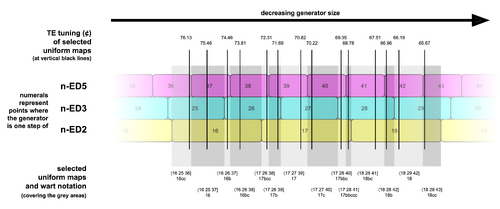# Uniform map

A uniform map is any map found by uniformly multiplying every entry of the just intonation point (JIP) for some prime limit [math]p[/math], log₂2 log₂3 log₂5 ... log₂[math]p[/math]], by the same positive real number before rounding them to integers.This visualizes EDO maps. We could call it the uniform map continuum. Generator size decreases to the right, so the EDO number goes up. Each colored cell is the closest approximation of steps to a prime for that generator size. We can see uniform maps as any set of step counts for primes found as perfectly vertical lines drawn through such a diagram. And integer uniform maps would be any such vertical line that is also drawn straight through a number which appears in the row for n-ED2.

What this means is that somewhere along the continuum of all possible equal-step tuning generator sizes, we can find one whose individually closest approximations for each of the primes is given by this map. In this sense, uniform maps are those that give reasonable tunings (further discussion of this idea may be found here). The map with the overall best tuning accuracy for an EDO will always be a uniform map. In many regular temperament theory contexts, uniform maps are the only maps used, such as in the famous projective tuning space diagrams from Paul Erlich's seminal A Middle Path paper, or the optimal GPV sequences given for many regular temperaments across the wiki.

For an example, choosing 17.1 as our uniform multiplier, we find the map 17.1·log₂2 log₂3 log₂5] = 17.1 27.103 39.705] which rounds to 17 27 40]. This is one of the many uniform maps for 17-EDO.

Every EDO has a finite number of possible uniform maps within a given prime limit, but an infinite number of uniform maps if no prime limit is given.

To find a uniform map for some [math]n[/math]-EDO, choose any multiplier that rounds to [math]n[/math] (anywhere from [math]n - 0.5[/math] to [math]n + 0.5[/math]). For example, 16.9 rounds to 17, so we could use that to find another example for 17-EDO. Uniformly multiplying 16.9·log₂2 log₂3 log₂5] = 16.9 26.786 39.241], which rounds to 17 27 39].

Subgroup uniform maps are also possible. For example, a uniform map on the 2.5.9/7 subgroup would be found by uniformly multiplying log₂2 log₂5 log₂(9/7)].

## Integer uniform map

A uniform map whose multiplier is an integer is called an integer uniform map. For example, we could use the integer 17 itself directly. So 17·log₂2 log₂3 log₂5] = 17 26.944 39.473], which also rounds to 17 27 39]. Every EDO has one integer uniform map.

## Simple map

Another name for an integer uniform map is a simple map. The two different terms provide two different ways of presenting the same object, which can be helpful in different contexts:

• In contexts pertaining to tuning accuracy, "simple map" works well. This is probably the more common context.
• In contexts pertaining to other uniform maps, "integer uniform map" works well.

To define "simple map" independently of "integer uniform map", it is a specific type of map used for EDOs. Every EDO has one simple map. The simple map for [math]n[/math]-EDO is found by taking the just intonation point (JIP) for some prime limit [math]p[/math], log₂2 log₂3 log₂5 ... log₂[math]p[/math]], multiplying it by [math]n[/math], then individually rounding entries to the nearest integer. For example, the simple map for 7-limit 19-EDO is 19·log₂2 log₂3 log₂5 log₂7] = 19 30.115 44.117 53.340] which rounds to 19 30 44 53].

So the simple map is not necessarily the best map for its EDO in terms of overall tuning accuracy, but it is the simplest map to calculate. The classic example of a simple map which is not the best map is 17p in the 5-limit, 17·log₂2 log₂3 log₂5] = 17 26.944 39.473] which rounds to 17 27 39]. The approximation of prime 5 is really bad here; it's about exactly halfway between 39 and 40 steps, but slightly below, which is why it rounds down. But it turns out that if we round up instead, using 40 steps to approximate prime 5, then the absolute errors in the primes remain about the same. However, the error in 5/3 is much less, because the error in 5 and the error in 3 are now in the same direction, canceling each other out, and so overall 17 27 40] has less error than 17 27 39].

## Vs. related terminology

A uniform map is the same thing as a generalized patent val, or GPV.

An integer uniform map or simple map is the same thing as a patent val.

As for the difference between "map" and "val", there is none, at least in most RTT cases; this is discussed here: Map.

Otherwise, the difference in terminology between uniform maps, integer uniform maps, patent vals, and GPVs, reflects an inversion in conceptual framing. For patent vals and GPVs, patent vals are considered the base case, and GPVs a generalization thereof, whereas for uniform maps and integer uniform maps, uniform maps are considered the base case and integer uniform maps a specialization thereof. There is an argument that uniform maps are the more fundamental and important concept to regular temperament theory and therefore that this framing is the superior of the two.